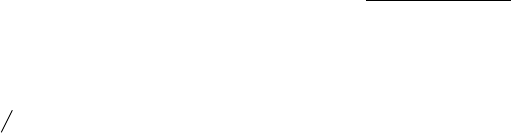Algebraic General Topology. Vol 1: Paperback / E-book || Axiomatic Theory of Formulas: Paperback / E-book

As an Amazon Associate I earn from qualifying purchases.Hyperfuncoids
by Victor Porton
Web: http://www.mathematics21.org
December 10, 2014
This is a rough partial draft.
1 Hyperfuncoids
Let A is an indexed family of sets.
Products are
Q
A for A 2
Q
A.
Problem 1. Is
d
FCD
a bijection from hyperfuncoids F¡ to:
1. prestaroids on A;
2. staroids on A ;
3. completary staroids on A?
If yes, is up
¡
deﬁning the inverse bijection?
If not, characterize the image of the function
d
FCD
deﬁned on F¡.
Alternatively (diﬀerently for the inﬁnite dimensional case!) deﬁne ¡ as the set of intersections
of sets with holes that is
Q
A n
Q
A where A v A. In other words, it is the set ¡
of complements
of elements of the set ¡.
Theorem 2. For every anchored relation f on powersets, f =
d
Anch(A)
up
¡
f.
Proof. We need to prove only f v
d
Anch(A)
up
¡
f.
Fix n 2 arity A. Let A 2
Q
(arity f)nfng
A
i
.
[TODO: Deﬁne the complement.]
Deﬁne g(A) =
Q
(arity f)nfng
A
i
× hf i
n
A
[
Q
(arity f)nfng
A
i
× 1
for A 2
Q
(arity f)nfng
A
i
.
Obviously g(A) 2 ¡
.
Let X 2
Q
(arity f)nfng
A
i
.
If 0 =/ X v A then hg(A)i
n
X = hf i
n
A w hf i
n
X.
If X v A then hg(A)i
n
X = 1.
So hG(A)i
n
w hf i
n
and thus G(A) w f .
For a given f, we have hg(A)i
n
A = hf i
n
A. Thus for every A 2
Q
(arity f)nfng
A
i
we have
hf i
n
A v
D
d
cStrd(A)
up
¡
f
E
n
A and so f v
d
Anch(A)
up
¡
f.
Corollary 3.
1. If f is a prestaroid, f =
d
pStrd(A)
up
¡
f.
2. If f is a staroid, f =
d
Strd(A)
up
¡
f.
3. If f is a completary staroid, f =
d
cStrd(A)
up
¡
f.
1